CONTACT

Name

e-mail×
×
×
×
×
×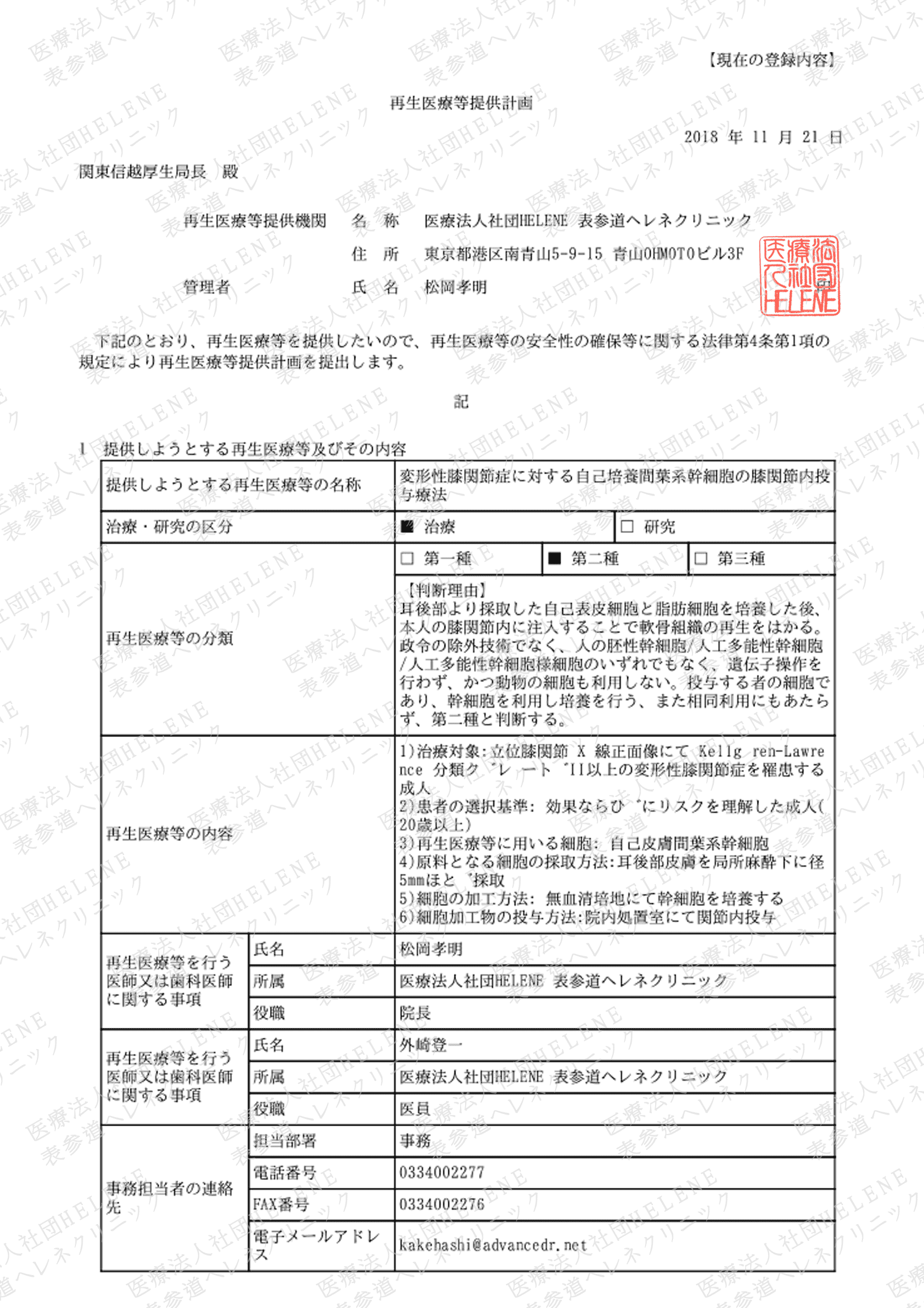×
×
×
×
×
×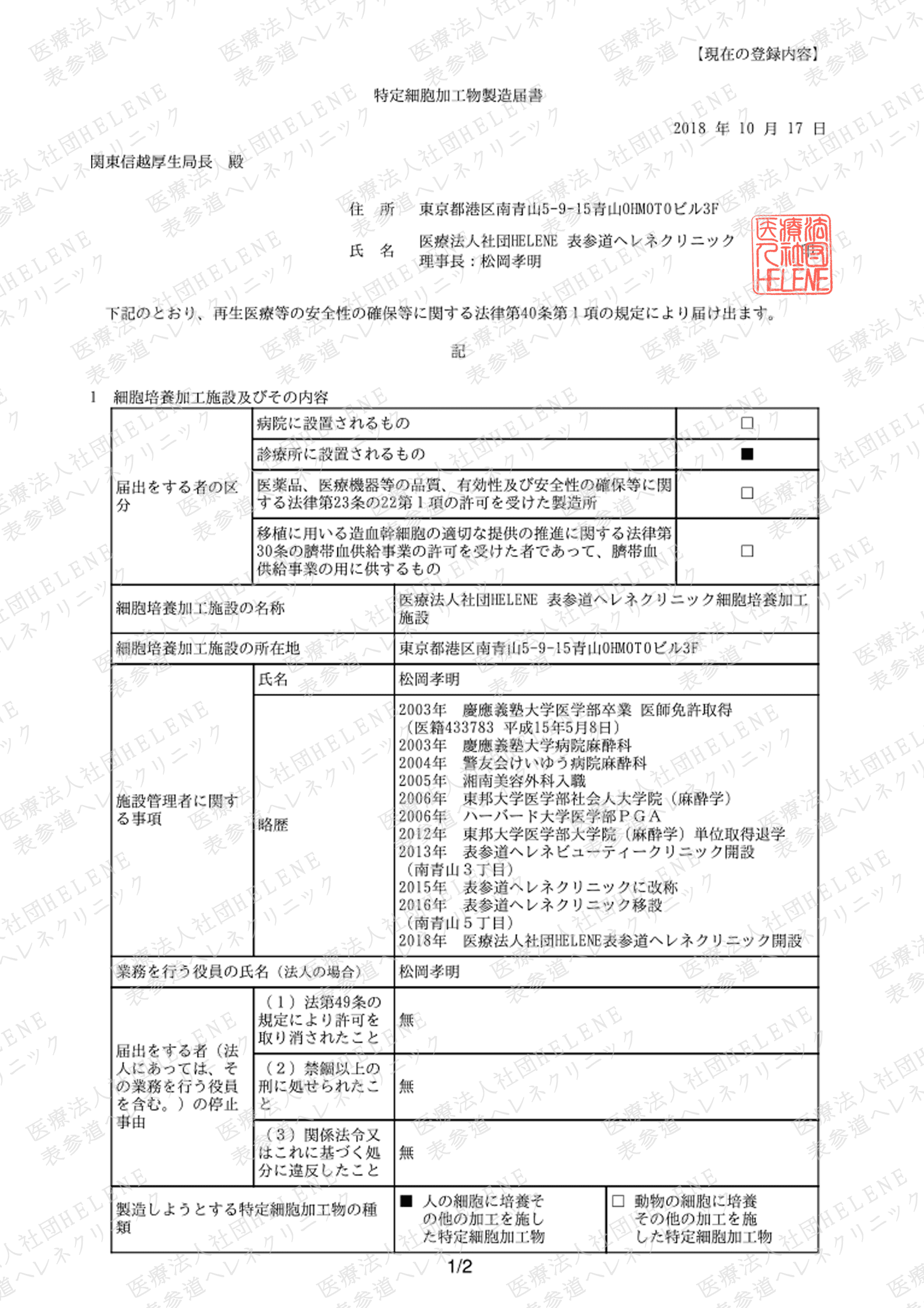×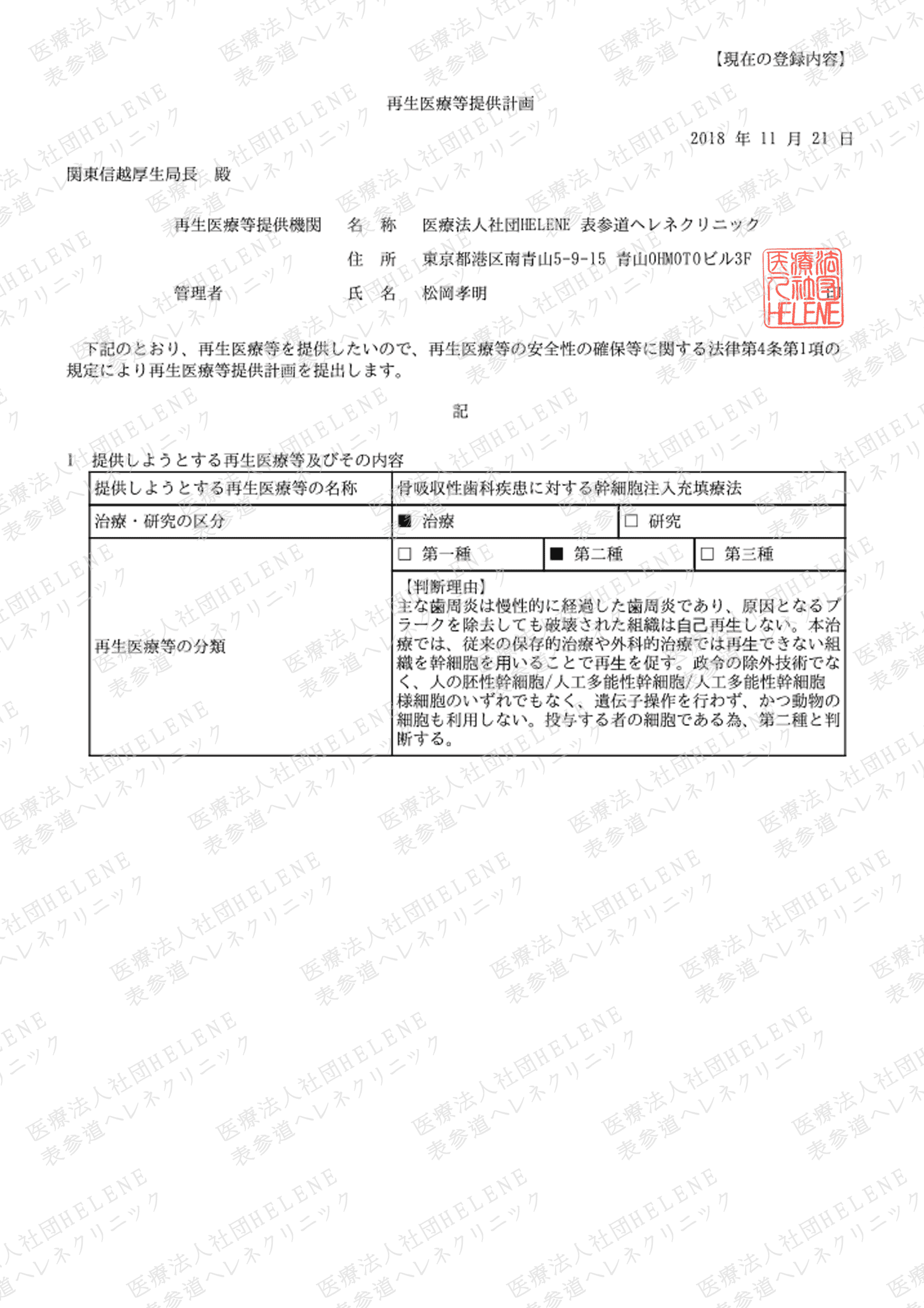×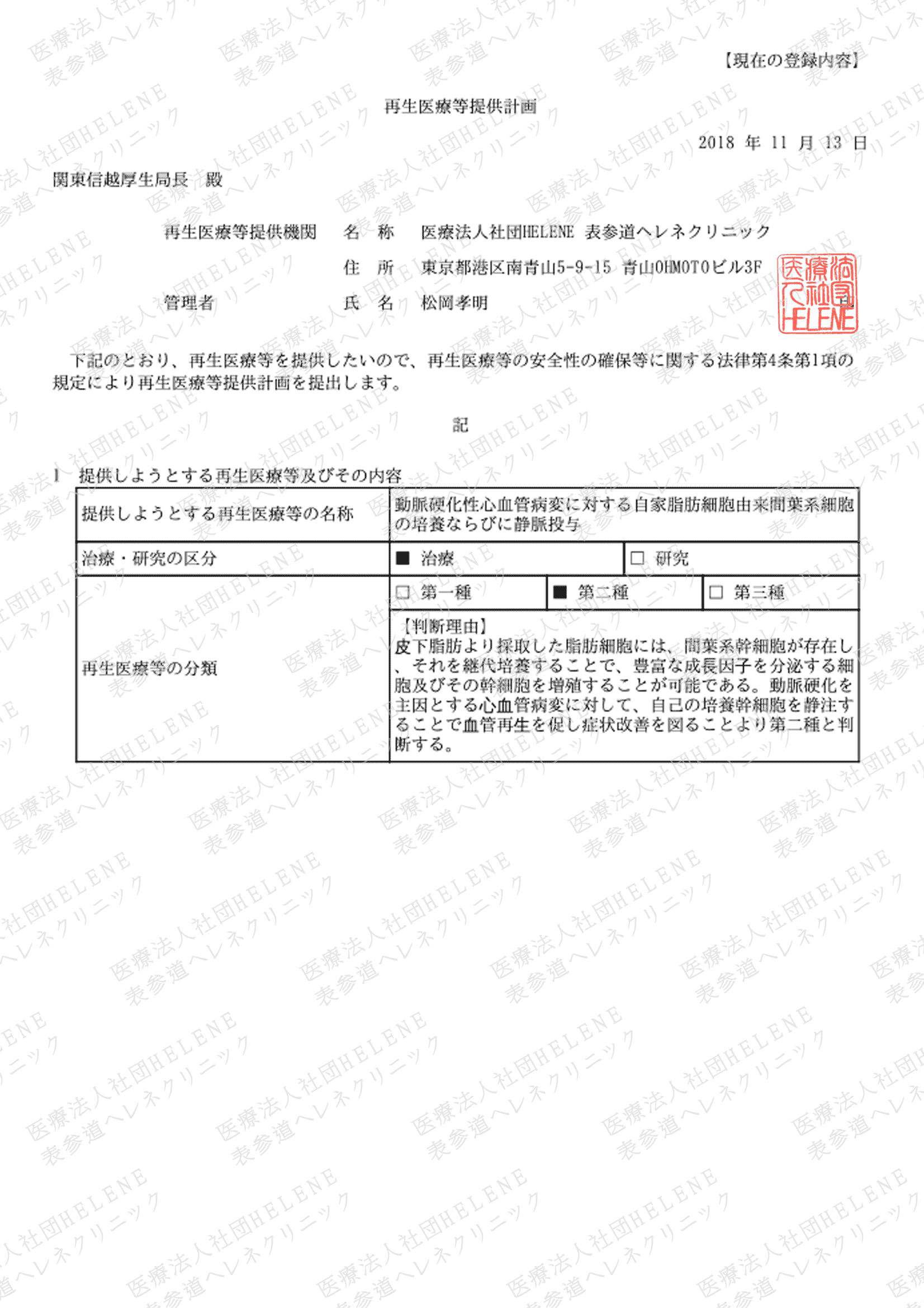×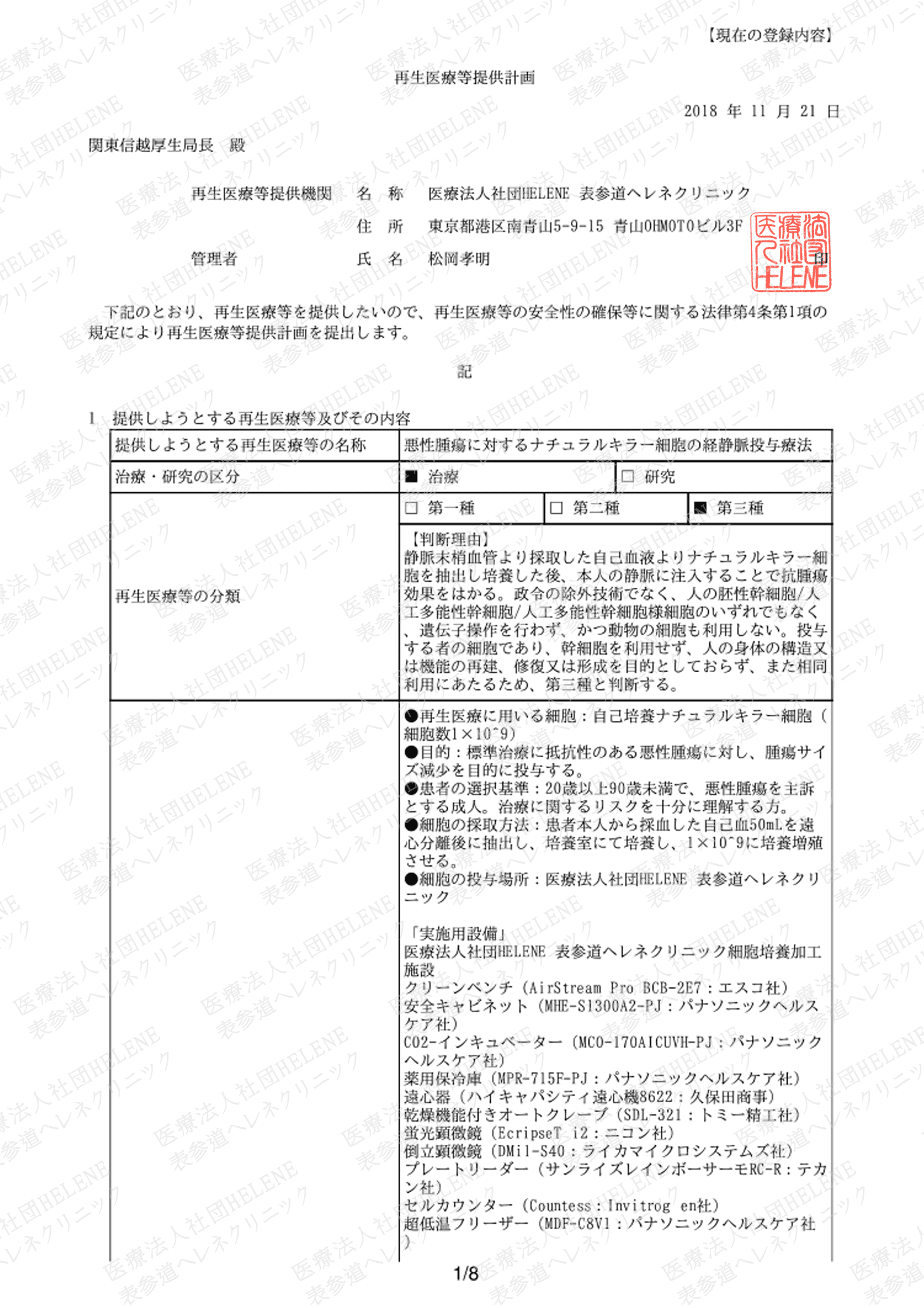×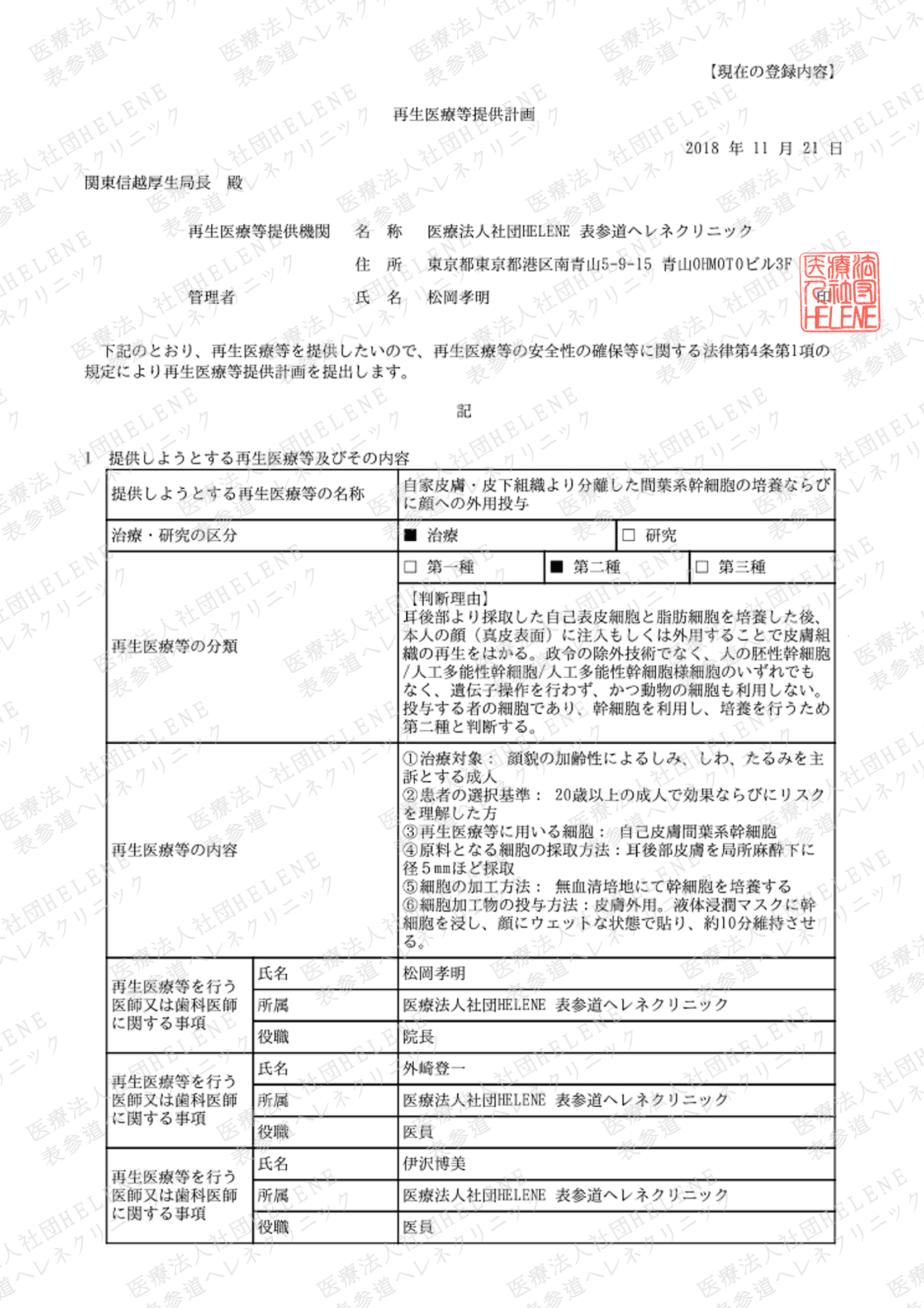×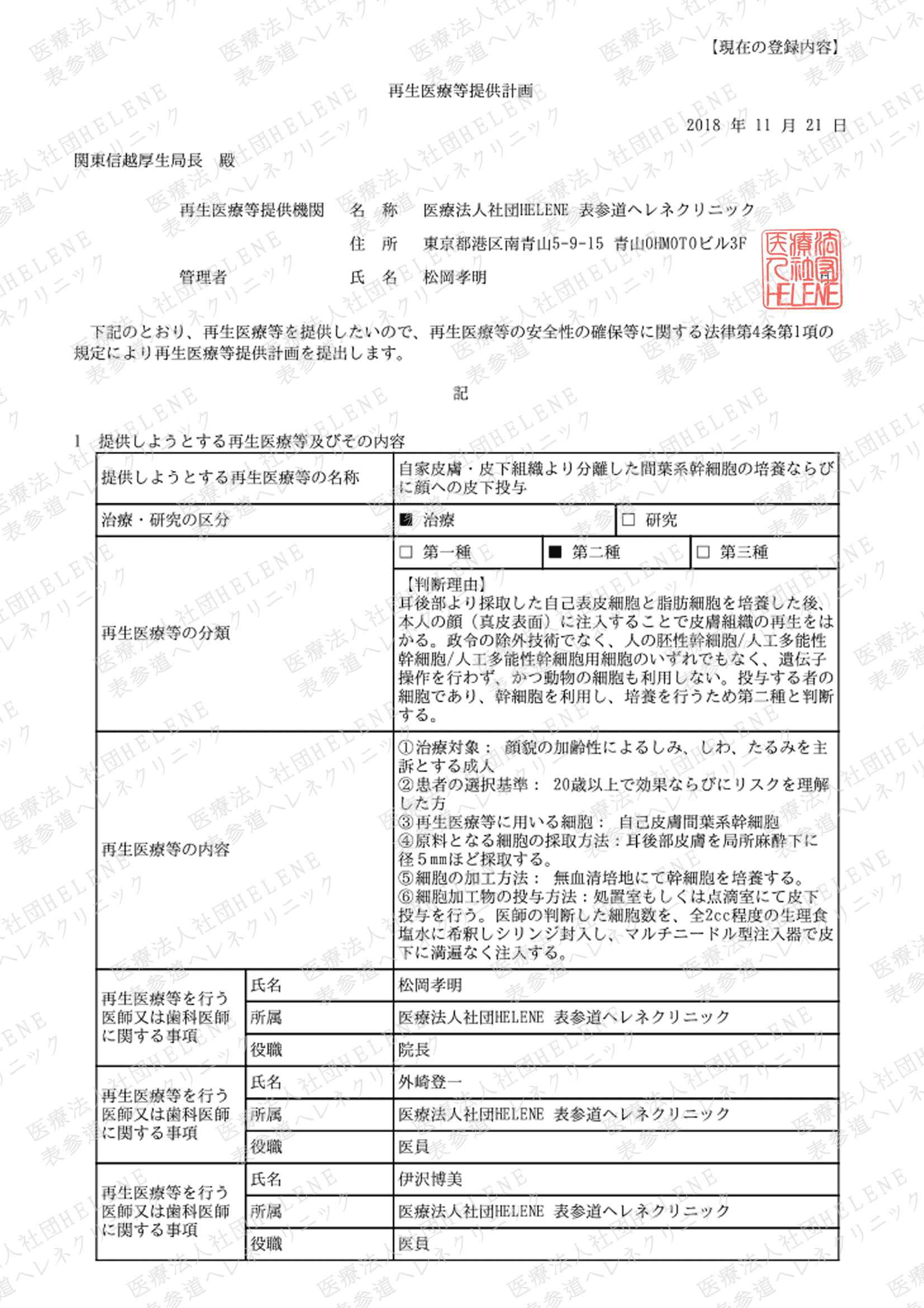×
×
×
×
×
×

×

×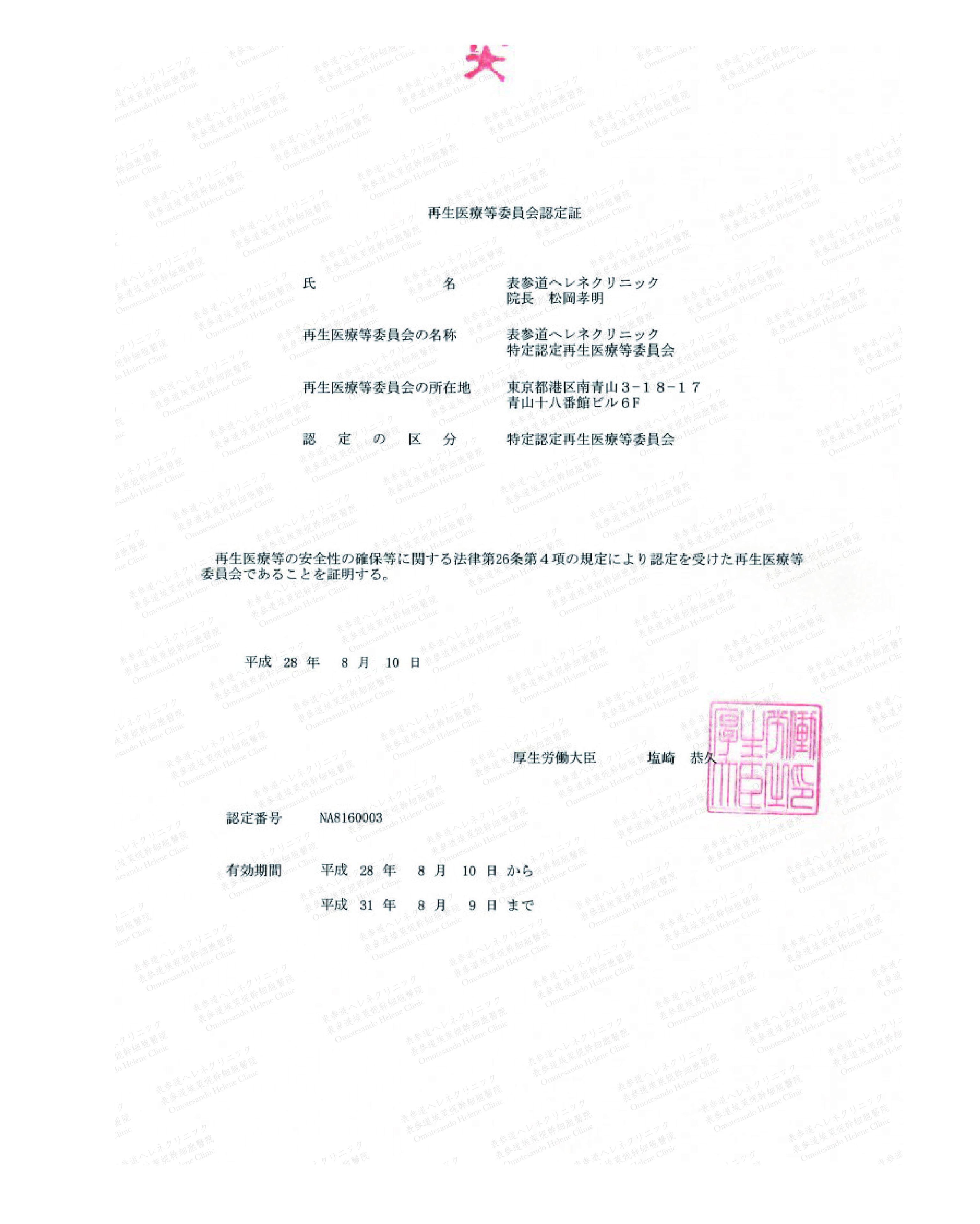×